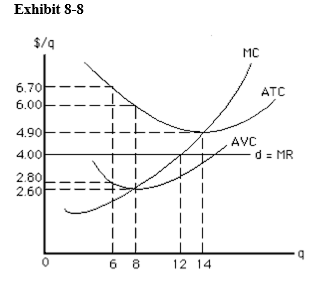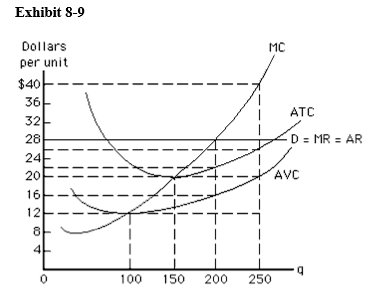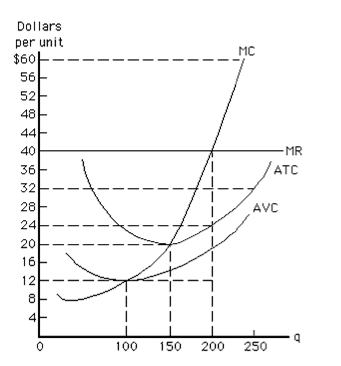# Chapter 8 Practice MC Flashcards

Set Details Share
created 6 years ago by melkojt
1,169 views
microecon
updated 6 years ago by melkojt
Page to share:
Embed this setcancel
COPY
code changes based on your size selection
Size:
X

1

Which of the following is not characteristic of perfect competition?

3. standardized products
4. fully informed buyers and sellers
5. free entry and exit of firms

2

Perfectly competitive firms respond to changing market conditions by varying their

1. price
2. output
3. market share
4. information

3

Which of the following is likely to be present in a perfectly competitive market?

1. patents
3. nonprice competition such as advertising
4. high capital costs
5. firms producing identical products

4

Firms in perfect competition have no control over

1. all of the following
2. where to operate on their average total cost curves
3. what price to charge
4. how many inputs to use
5. how much to produce

5

Perfectly competitive firms are price takers because

1. all small firms must take the price set by the largest firm in the market
2. firms take the price that government determines is a "fair" price
3. each firm is small and goods are perfect substitutes for one another
4. free entry and exit in the short run creates a constant market price in the long run
5. high barriers to entry force firms to compete by charging lower prices than other firms in the industry

6

The price charged by a perfectly competitive firm is determined by

1. each individual firm
2. a group of firms acting together as a cartel
3. market demand and market supply
4. the firm's total costs alone
5. the firm's average variable cost

7

The demand curve for the output of a perfectly competitive firm is

1. perfectly inelastic
2. perfectly elastic
3. unit elastic
4. downward sloping
5. nonlinear

8

Suppose the equilibrium price in a perfectly competitive industry is \$100 and a firm in the industry charges

1. The firm will not sell any of its output.
2. The firm will sell more output than its competitors.
3. The firm's profits will increase.
4. The firm's revenue will increase.
5. The firm will gradually take over the entire industry.

9

Commodity products are

1. rare and expensive
3. highly differentiated
4. uniform or standardized
5. ones without impurities

10

Marginal revenue is defined as

1. total revenue divided by quantity
2. total revenue minus total cost
3. the change in total revenue divided by the change in quantity
4. the change in total revenue divided by quantity
5. the change in total revenue

11

A perfectly competitive firm's profit per unit of output equals

1. price minus average variable cost
2. price minus marginal cost
3. total revenue minus total cost
4. price times quantity
5. price minus average total cost

12If the price-taking firm in Exhibit 8-8 is currently producing 6 units, then to maximize profit in the short run, it should

1. keep producing 6 units
2. increase production to 12 units
3. increase production to 14 units
4. increase production to 8 units
5. shut down

13At the profit-maximizing output level, the firm represented in Exhibit 8-9 experiences

1. a loss of \$3,200
2. a profit of \$1,600
3. a profit of \$1,200
4. zero profit or loss
5. a loss of \$800

14At the profit-maximizing output level, the firm represented in Exhibit 8-10 experiences

1. a loss of \$3,200
2. a profit of \$6,000
3. a profit of \$3,200
4. zero profit or loss
5. a loss of \$6,000

15

In the short run, if a firm shuts down, its loss is equal to

1. \$0
2. its variable costs
3. its fixed costs
4. fixed costs minus variable costs
5. fixed costs minus total revenue

16

In the short run, a perfectly competitive ball bearing manufacturer will continue to produce at a loss if

1. it is covering all of its fixed cost
2. it is covering all of its variable cost plus part of its fixed cost
3. variable cost is less than fixed cost
4. fixed cost is zero
5. fixed cost is minimized

17

Claude's Copper Clappers sells clappers for \$40 each in a perfectly competitive market. At its present rate of output, Claude's marginal cost is \$39, average variable cost is \$45, and average total cost is \$60. To improve his profit/loss situation, Claude should

1. increase output
2. reduce output but not to zero
3. maintain the present rate of output
4. shut down
5. raise the price

18

If price is less than its minimum average variable cost, a perfectly competitive firm that continues to produce in the short run

1. cannot cover any of its variable cost
2. incurs a loss greater than its fixed cost
3. can cover all of its fixed cost and some of its variable cost
4. can cover all of its variable cost and some of its fixed cost
5. can cover both its fixed costs and its variable cost

19

In the short run, a firm will produce a positive amount of output as long as

1. P > AVC at some output level
2. P > MC at some output level
3. P < AVC at some output level
4. AVC < ATC at some output level
5. FC > TR at some output level

20

Many country inns shut down in the off-season because

1. the off-season market price falls below average total cost
2. the off-season market price can't cover their average fixed cost
3. the off-season revenue can't cover variable cost
4. the off-season price is below the marginal cost of providing a room
5. innkeepers are interested in maximizing revenue

21

A perfectly competitive firm will produce at an economic loss (negative profit) in the short run rather than discontinue production if there is a rate of output at which price

1. exceeds average variable cost
2. exceeds average fixed cost
3. exceeds average total cost
4. exceeds marginal revenue
5. equals marginal cost

22

The price that represents the shutdown point for a perfectly competitive firm is the

1. highest point on the marginal cost curve
2. lowest point on the marginal cost curve
3. highest point on the average variable cost curve
4. lowest point on the average variable cost curve
5. lowest point on the average total cost curve

23

The perfectly competitive firm's short-run supply curve is the same as the

1. supply curve of all other firms in the industry
2. upward-sloping portion of its marginal cost curve
3. upward-sloping portion of its marginal cost curve at or above minimum average variable cost
4. upward-sloping portion of its average variable cost curve
5. market demand curve

24

A perfectly competitive firm in the short run determines its quantity supplied at various prices by using

1. the portion of its marginal cost curve rising above its average total cost curve
2. the portion of its marginal cost curve rising above its average variable cost
3. its average variable cost curve
4. its average total cost curve
5. the portion of its average variable cost curve rising above its average fixed cost curve

25

Which of the characteristics of perfect competition assures that economic profit will be zero in the long run?

1. Each firm is small relative to the market.
3. Goods produced in the market are homogeneous.
4. Each firm is a price taker.
5. There is easy entry and exit in the market.

26

Long-run equilibrium for a perfectly competitive firm occurs when

1. P = MC = MR = ATC
2. MC = MR = AFC = ATC
3. MC = MR = P > ATC
4. P > MC > MR > ATC
5. TR > TC

27

Firms in perfect competition will leave the industry if they

1. suffer short-run losses
2. suffer losses, even if they are covering variable costs in the short run
3. suffer long-run losses
4. earn a normal profit
5. earn a zero economic profit

28

The motivating force behind an increase in supply in a long-run adjustment to equilibrium is

1. lower prices
2. economic profits that are present in the short run
3. higher profit expectations among owners of firms in the industry, triggered by increased prices
4. normal profits witnessed by individuals outside the industry that trigger entry
5. the decreases in average cost that can be obtained through economies of scale

29

If a perfectly competitive firm is operating in long-run equilibrium and market demand suddenly falls, the short-run result will be

1. greater economic profit
2. a normal profit
3. lower average total cost
4. lower average variable cost
5. an economic loss

30

Firms achieve productive efficiency in the long run by

1. striving to minimize fixed cost
2. striving to maximize revenue
3. producing at their minimum long-run average cost
4. producing at their minimum long-run marginal cost
5. producing the output consumers want most

31

Productive efficiency occurs in markets when

1. goods are produced at the lowest possible average total cost
2. goods are produced at the lowest average variable cost
3. goods are produced at the lowest marginal cost
4. goods are produced at the lowest average fixed cost
5. the economy is producing maximum quantity of goods and services it can

32

To achieve allocative efficiency, firms

1. strive to minimize fixed costs
2. strive to maximize profits
3. produce at their minimum long-run marginal cost
4. produce at their minimum long-run marginal cost
5. produce the output consumers want most

33

Allocative efficiency occurs in markets when

1. marginal benefit and marginal cost for the last unit sold are equal
2. resources can be reallocated to increase the value of total output
3. goods are produced at the minimum of average total cost
4. goods are distributed evenly among consumers
5. government establishes price ceilings below the market price

34

Allocative efficiency means that

1. firms have maximized production
2. all mutually beneficial trades have taken place
3. the next unit sold will increase total surplus
4. producer surplus is maximized
5. no mutually beneficial trades have occurred

35

When market exchange occurs voluntarily in a competitive market

1. choice incurs no opportunity cost
2. the sum of consumer surplus and producer surplus is maximized
3. both consumer surplus and producer surplus are eliminated
4. buyers benefit at the expense of producers
5. the exchange confers no net benefit to the participants